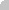property Column.ComputedField as String
Retrieves or sets a value that indicates the formula of the computed column.Type Description String A String expression that indicates the formula to compute the field/cell. The formula is applied to all cells in the column with the CellCaptionFormat property on exText ( the exText value is by default ).
A computed field or cell displays the result of an arithmetic formula that may include operators, variables and constants. By default, the ComputedField property is empty. If the the ComputedField property is empty, the property have no effect. If the ComputedField property is not empty, all cells in the column, that have the CellCaptionFormat property on exText, uses the same formula to display their content. For instance, you can use the CellCaptionFormat property on exHTML, for cells in the column, that need to display other things than column's formula, or you can use the CellCaptionFormat property on exComputedField, to change the formula for a particular cell. Use the FormatColumn property to format the column. Use the CellCaptionFormat property to change the type for a particular cell. Use the CellCaption property to specify the cell's content. For instance, if the CellCaptionFormat property is exComputedField, the Caption property indicates the formula to compute the cell's content.  The Def(exCellCaptionFormat) property is changed to exComputedField, each time the ComputeField property is changed to a not empty value. If the ComputedField property is set to an empty string, the Def(exCellCaptionFormat) property is set to exText. Call the Refresh method to force refreshing the control.

The expression supports cell's identifiers as follows:

• %0, %1, %2, ... specifies the value of the cell in the column with the index 0, 1 2, ... The CellCaption property specifies the cell's value. For instance, "%0 format ``" formats the value on the cell with the index 0, using current regional setting, while "int(%1)" converts the value of the column with the index 1, to integer.

This property/method supports predefined constants and operators/functions as described here.

Samples:

1. "1", the cell displays 1
2. "%0 + %1", the cell displays the sum between cells in the first and second columns.
3. "%0 + %1 - %2", the cell displays the sum between cells in the first and second columns minus the third column.
4. "(%0 + %1)*0.19", the cell displays the sum between cells in the first and second columns multiplied with 0.19.
5. "(%0 + %1 + %2)/3", the cell displays the arithmetic average for the first three columns.
6. "%0 + %1 < %2 + %3", displays 1 if the sum between cells in the first  two columns is less than the sum of third and forth columns.
7. "proper(%0)'" formats the cells by capitalizing first letter in each word
8. "currency(%1)'" displays the second column as currency using the format in the control panel for money
9. "len(%0) ? currency(dbl(%0)) : ''" displays the currency only for not empty/blank cells.
10. "int(date(%1)-date(%2)) + 'D ' + round(24*(date(%1)-date(%2) - floor(date(%1)-date(%2)))) + 'H''" displays interval between two dates in days and hours, as xD yH
11. "2:=((1:=int(0:= date(%1)-date(%0))) = 0 ? '' : str(=:1) + ' day(s)') + ( 3:=round(24*(=:0-floor(=:0))) ? (len(=:2) ? ' and ' : '') + =:3 + ' hour(s)' : '' )" displays the interval between two dates, as x day(s) [and y hour(s)], where the x indictaes the number of days, and y the number of hours. The hour part is missing, if 0 hours is displayed, or nothing is displayed if dates are identical.